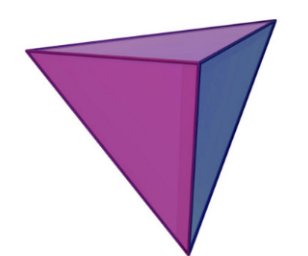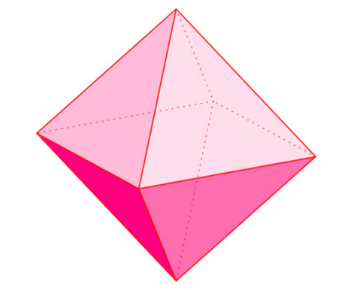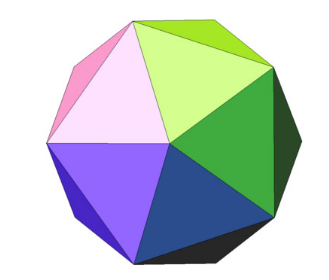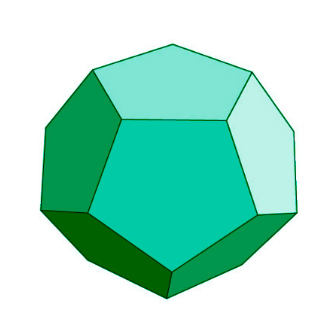Jet Set Go! All about Aeroplanes Jet Set Go! All about Aeroplanes

# Euler’s Formula For Polyhedra

Euler’s formula for Polyhedra gives the basic condition for any three-dimensional shape being polyhedra. Polyhedra, plural of a polyhedron, is a three-dimensional closed figure whose faces are flat and polygonal, edges are made of straight lines and corners are sharp. Example of polyhedrons is pyramids, tetrahedrons, etc.

In 1758, a Swiss mathematician Leonhard Euler gave a formula for convex polyhedrons relating their faces, edges and vertices. This became the condition for any three-dimensional figure being a convex polyhedron.

## What is the Euler’s Formula for Polyhedrons?

Despite the straightforward definition of polyhedra, it was quite difficult to categorise them explicitly. Euler’s characteristic equation gave an important condition for the surfaces of polyhedrons. The characteristic equation is given as

χ = V – E + F, where

V is the number of vertices of the polyhedra,

E is the number of edges, and

F is the number of faces of polyhedra.

For convex polyhedrons, χ = 2. Thus we have Euler’s formula for polyhedrons as

 V – E + F = 2.

Let us check this formula for the five Platonic solids:

 S.No. Polyhedron Name Shape Vertices V Edges E Faces F Euler’s Formula V – E + F = 2 1. Tetrahedron4 6 4 4 – 6 + 4 = –2 + 4 = 2 2. Hexahedron or Cube8 12 6 8 – 12 + 6 = – 4 + 6 = 2 3. Octahedron6 12 8 6 – 12 + 8 = – 6 + 8 = 2 4. Icosahedron12 30 20 12 – 30 + 20 = –18 + 20 = 2 5. Dodecahedron20 30 12 20 – 30 + 12 = – 10 + 12 = 2.

## Proof of Euler’s Formula

We shall prove Euler’s formula using graph theory.

Refer

We prove the formula by applying induction on edges by considering the polyhedra as a simply connected planar graph G with v vertices, e edges and f faces.

If G has zero number of edges, that is e = 0. The graph will have a single with f = 1. Then, by the Euler’s formula V – E + F = 1 – 0 + 1 = 2. Thus, it satisfies Euler’s formula.

Induction step: Let the Euler’s formula is applicable for a graph with n edges.

Let G be a graph with n + 1 edges.

Case I: If G is a tree and does not contain any cycle. It can be easily proven by induction for trees with any number of edges.

Case II: If G is not a tree and contains atleast one cycle. Choose an edge e1 in G which divides the given region into two different parts and remove that edge e1 to get another graph G’’.

Faces in G > Faces in G’’

Now, G has n + 1 edges, then G’’ has n edges so by the hypothesis G’’ satisfies the Euler’s formula. For G’’, V’ – E’ + F’ = 2 where V’ = v, E’ = e – 1 and F’ = f – 1.

Now, substituting these values, we get

V’ – E’ + F’ = 2

⇒ v – e + 1 + f – 1 = 2

⇒ v – e + f = 2

Hence, Euler’s formula is applicable for n + 1 edges.

This proves the Euler’s formula.

## Solved Examples on Euler’s Formula for Polyhedra

Example 1:

Prove the Euler’s formula for a triangular prism.

Solution:

Number of vertices of a triangular prism = 6

Number of edges of a triangular prism = 9

Number of faces of a triangular prism = 5

By the Euler’s formula

V – F + E = 6 – 9 + 5 = – 3 + 5 = 2

⇒ V – F + E = 2

Thus, Euler’s formula is satisfied for a triangular prism.

Example 2:

Is cuboid a polyhedron?

Solution:

If cuboid is a polyhedron then it must satisfy Euler’s formula for polyhedra.

Number of edges, e = 12

Number of vertices, v = 8

Number of faces, f = 6

By the Euler’s formula:

v – e + f = 8 – 12 + 6 = –4 + 6 = 2.

⇒ v – e + f = 2.

which satisfies the Euler’s formula. Hence, the cuboid is a polyhedron.

## Practice Questions:

1. Is it possible to have a polyhedron with three triangular faces?

2. Complete the following table:

 S.No. Faces Edges Vertices 1. 6 12 … 2. … 30 12 3. 12 … 20

### Related Videos## Frequently Asked Questions on Euler’s Formula

### What is Euler’s formula for solid shapes?

Euler’s characteristic formula for polyhedrons is χ = V – E + F and for convex polyhedrons χ = 2.

### What is the formula for polyhedron?

Euler’s formula for polyhedra is V – E + F = 2 where V is the number of vertices, E is the number of edges and F is the number of faces of a polyhedron.

### Does Euler’s formula work for all polyhedra?

There are few constraints for the applicability of Euler’s formula:

• The polyhedron must not have holes in it.
• It must not intersect itself.
• It must not be made by connecting two polygons.

### What is the Euler’s formula for a polyhedra for class 8?

For a polyhedron, the Euler’s formula is given by V – E + F = 2 where V is the number of vertices, E is the number edges and F is the number of faces of a polyhedron.

### Is a cylinder a polyhedron?

No, a cylinder is not a polyhedron.

To learn more such topics on three-dimensional shapes with video lessons and personalised notes, download the BYJU’S – The Learning App today and register yourself for the journey of learning.